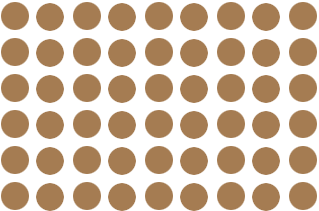Name: Sergio Who is asking: Parent Level of the question: Elementary Question: Hi my son Anthony is in Third Grade and the question on his homework that we're having trouble answering is the following: Name two smaller arrays you can use to find the product. 6 x 9 = 54 What are they exactly asking? Which is the correct answer? Thank you in advance Sergio Hi Sergio and Anthony, An array is a rectangular arrangement. Think of something like 54 pennies. You can arrange them into 6 rows and 9 columns. This is a 6 by 9 array. (Mathematicians always state the number of rows first and then the number of columns.)I think what the question is asking is for you to arrange the 54 pennies into two smaller arrays. There are many different answers. One is to make a 4 by 7 array and a 13 by 2 array, since 47 = 28 and 132 = 26 and 28 + 26 = 54. I am not sure what Anthony's teacher means by smaller. Both of these arrays have fewer pennies but the second has more rows than the original 6 by 9 array. Can you find two arrays where both have fewer than 6 rows and fewer than 9 columns? Penny Hi, By breaking down the numbers you can make it easier to find answers. So that 69 = 54 is the same thing as (39 = 27) + (39 = 27). 612 = is the same thing as (66 = 36) + (66 = 36). It makes it easier to find the answer if you don’t know that 612 = 72. If you don’t know that 6 x 6 is 36 you can break it down even further so that it looks like (36 = 18) + (36 = 18) + (36 = 18) + (36 = 18) = 72. Hope this helps. Diane